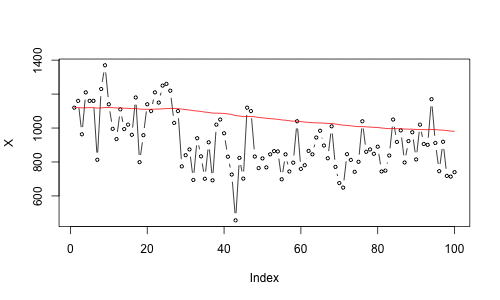# Holt-Winters with a Quantile Loss Function

January 8, 2018
By

Want to share your content on R-bloggers? click here if you have a blog, or here if you don't.

Exponential Smoothing is an old technique, but it can perform extremely well on real time series, as discussed in Hyndman, Koehler, Ord & Snyder (2008)),

when Gardner (2005) appeared, many believed that exponential smoothing should be disregarded because it was either a special case of ARIMA modeling or an ad hoc procedure with no statistical rationale. As McKenzie (1985) observed, this opinion was expressed in numerous references to my paper. Since 1985, the special case argument has been turned on its head, and today we know that exponential smoothing methods are optimal for a very general class of state-space models that is in fact broader than the ARIMA class.

Furthermore, I like it because I think it has nice pedagogical features. Consider simple exponential smoothing, $$L_{t}=\alpha Y_{t}+(1-\alpha)L_{t-1}$$ where $\alpha\in(0,1)$ is the smoothing weight. It is locally constant, in the sense that ${}_{t}\hat Y_{t+h} = L_{t}$

 1 2 3 4 5 6 7 8 9 10 11   library(datasets) X=as.numeric(Nile) SimpleSmooth = function(a){ T=length(X) L=rep(NA,T) L=X for(t in 2:T){L[t]=a*X[t]+(1-a)*L[t-1]} return(L) } plot(X,type="b",cex=.6) lines(SimpleSmooth(.2),col="red")When using the standard R function, we get

 1 2 3  hw=HoltWinters(X,beta=FALSE,gamma=FALSE, l.start=X) hw$alpha  0.2465579 Of course, one can replicate that optimal value  1 2 3 4 5 6 7 8 9 10 11 12  V=function(a){ T=length(X) L=erreur=rep(NA,T) erreur=0 L=X for(t in 2:T){ L[t]=a*X[t]+(1-a)*L[t-1] erreur[t]=X[t]-L[t-1] } return(sum(erreur^2)) } optim(.5,V)$par  0.2464844

Here, the optimal value for $\alpha$ is the one that minimizes the one-step prediction, for the $\ell_2$ loss function, i.e. $$\sum_{t=2}^n(Y_t-{}_{t-1}\hat Y_t)^2$$ where here ${}_{t-1}\hat Y_t = L_{t-1}$. But one can consider another loss function, for instance the quantile loss function, $$\ell_{\tau}(\varepsilon)=\varepsilon(\tau-\mathbb{I}_{\varepsilon\leq 0})$$. The optimal coefficient is then obtained using

 1 2 3 4 5 6 7 8 9 10 11 12 13 14  HWtau=function(tau){ loss=function(e) e*(tau-(e<=0)*1) V=function(a){ T=length(X) L=erreur=rep(NA,T) erreur=0 L=X for(t in 2:T){ L[t]=a*X[t]+(1-a)*L[t-1] erreur[t]=X[t]-L[t-1] } return(sum(loss(erreur))) } optim(.5,V)$par } Here is the evolution of $\alpha^\star_\tau$ as a function of $\tau$ (the level of the quantile considered).  1 2 3 4  T=(1:49)/50 HW=Vectorize(HWtau)(T) plot(T,HW,type="l") abline(h= hw$alpha,lty=2,col="red")Note that the optimal $\alpha$ is decreasing with $\tau$. I wonder how general this result can be…

Of course, one can consider more general exponential smoothing, for instance the double one, with $$L_t=\alpha Y_t+(1-\alpha)[L_{t-1}+B_{t-1}]$$and$$B_t=\beta[L_t-L_{t-1}]+(1-\beta)B_{t-1}$$so that the prediction is now ${}_{t}\hat Y_{t+h} = L_{t}+hB_t$ (it is now locally linear – and no longer constant).

 1 2 3 4 5 6 7  hw=HoltWinters(X,gamma=FALSE,l.start=X) hw$alpha alpha 0.4200241 hw$beta beta 0.05973389

The code to compute the smoothed series is the following

 1 2 3 4 5 6 7 8 9  DoubleSmooth = function(a,b){ T=length(X) L=B=rep(NA,T) L=X; B=0 for(t in 2:T){ L[t]=a*X[t]+(1-a)*(L[t-1]+B[t-1]) B[t]=b*(L[t]-L[t-1])+(1-b)*B[t-1] } return(L+B) }

Here also it is possible to replicate R using the $\ell_2$ loss function

 1 2 3 4 5 6 7 8 9 10 11 12 13 14 15  V=function(A){ a=A b=A T=length(X) L=B=erreur=rep(NA,T) erreur=0 L=X; B=X-X for(t in 2:T){ L[t]=a*X[t]+(1-a)*(L[t-1]+B[t-1]) B[t]=b*(L[t]-L[t-1])+(1-b)*B[t-1] erreur[t]=X[t]-(L[t-1]+B[t-1]) } return(sum(erreur^2)) } optim(c(.5,.05),V)$par  0.41904510 0.05988304 (up to numerical optimization approximation, I guess). But here also, a quantile loss function can be considered  1 2 3 4 5 6 7 8 9 10 11 12 13 14 15 16 17  HWtau=function(tau){ loss=function(e) e*(tau-(e<=0)*1) V=function(A){ a=A b=A T=length(X) L=B=erreur=rep(NA,T) erreur=0 L=X; B=X-X for(t in 2:T){ L[t]=a*X[t]+(1-a)*(L[t-1]+B[t-1]) B[t]=b*(L[t]-L[t-1])+(1-b)*B[t-1] erreur[t]=X[t]-(L[t-1]+B[t-1]) } return(sum(loss(erreur))) } optim(c(.5,.05),V)$par }

and we can plot those values on a graph

 1 2 3 4 5 6  T=(1:49)/50 HW=Vectorize(HWtau)(T) plot(HW[1,],HW[2,],type="l") abline(v= hw$alpha,lwd=.4,lty=2,col="red") abline(h= hw$beta,lwd=.4,lty=2,col="red") points(hw$alpha,hw$beta,pch=19,col="red")(with $\alpha$ on the $x$-axis, and $\beta$ on the $y$-axis). So here, it is extremely simple to change the loss function, but so far, it should be done manually. Of course, one do it also for the seasonal exponential smoothing model.

R-bloggers.com offers daily e-mail updates about R news and tutorials about learning R and many other topics. Click here if you're looking to post or find an R/data-science job.
Want to share your content on R-bloggers? click here if you have a blog, or here if you don't.